For question 5 on page 242(Angles, Triangle and Polygons), could you explain why the triangle was put into 60,30,90 right triangle and how you came up with b/2 *square root of 3 as the height?

Sure. The question is: “The area of a regular hexagon is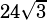. What is its perimeter?”

The solution notes that a regular hexagon is made up of six equilateral triangles, and goes from there. I think you’re stuck on this figure from the solution?

Let’s break it down.

We’re working from the given that the larger triangle is equilateral, which explains why all the sides have length. We know equilateral triangles have three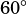angles.

Therefore, when we drop a vertical line from the top, we create a right triangle with one of its acute angles being.

If one angle isand another angle is a right angle, then the third angle must be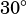.

So we know we have a 30-60-90 and we know its hypotenuse has length.

In a 30-60-90, we know the sides are generally, from smallest to largest,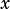,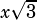, and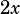. The hypotenuse ofwe have here corresponds to, so the short leg must be half that,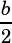. From there, we know the long leg is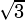times the short leg, or.

Does that help?